# Verbal Reasoning - Dice

### Exercise :: Dice - Dice 3

The figure given on the left hand side in each of the following questions is folded to form a box. Choose from the alternatives (1), (2), (3) and (4) the boxes that is similar to the box formed.
1.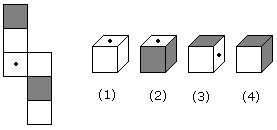A. 2 and 3 only B. 1, 3 and 4 only C. 2 and 4 only D. 1 and 4 only

Explanation:

No answer description available for this question. Let us discuss.

2.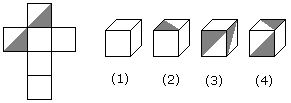A. 1, 2 and 4 only B. 3 and 4 only C. 1 and 2 only D. 1, 2 and 3 only

Explanation:

No answer description available for this question. Let us discuss.

3.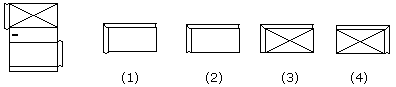A. 1 only B. 2 only C. 3 only D. 4 only

Explanation:

No answer description available for this question. Let us discuss.

4.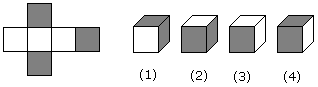A. 1 and 3 only B. 2 and 4 only C. 2 and 3 only D. 1, 2, 3 and 4

Explanation:

No answer description available for this question. Let us discuss.

5.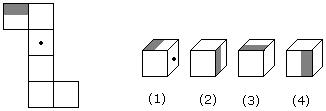A. 1 and 2 only B. 2 and 3 only C. 1, 2, 3 and 4 D. 2 and 4 only